# Mean State

Period Mean (original grids) [Watt m-2]
Model Period Mean (intersection) [Watt m-2]
Model Period Mean (complement) [Watt m-2]
Benchmark Period Mean (intersection) [Watt m-2]
Benchmark Period Mean (complement) [Watt m-2]
Bias [Watt m-2]
RMSE [Watt m-2]
Phase Shift [months]
Bias Score 
RMSE Score 
Seasonal Cycle Score 
Spatial Distribution Score 
Interannual Variability Score 
Overall Score 
Benchmark [-] -56.8
CRUNCEPv7 [-] -56.5 -56.4 0.00 -57.0 -52.3 1.23 14.0 2.12 0.59 0.46 0.68 0.99 0.55 0.62
GSWP3v1 [-] -66.5 -66.5 0.00 -57.0 -52.3 -9.56 16.5 1.67 0.44 0.46 0.76 0.97 0.82 0.65
WATCH [-] -53.9 -53.6 0.00 -55.9 -52.5 1.71 11.5 1.16 0.63 0.51 0.84 0.99 0.74 0.71
Period Mean (original grids) [Watt m-2]
Model Period Mean (intersection) [Watt m-2]
Model Period Mean (complement) [Watt m-2]
Benchmark Period Mean (intersection) [Watt m-2]
Benchmark Period Mean (complement) [Watt m-2]
Bias [Watt m-2]
RMSE [Watt m-2]
Phase Shift [months]
Bias Score 
RMSE Score 
Seasonal Cycle Score 
Spatial Distribution Score 
Interannual Variability Score 
Overall Score 
Benchmark [-] -87.0
CRUNCEPv7 [-] -90.0 -90.7 0.00 -87.4 -58.4 -2.82 19.4 0.297 0.76 0.58 0.97 0.91 0.53 0.72
GSWP3v1 [-] -97.3 -98.0 0.00 -87.4 -58.4 -10.2 22.1 0.309 0.68 0.59 0.97 0.81 0.72 0.73
WATCH [-] -80.4 -81.1 0.00 -85.5 -58.4 4.59 15.2 0.174 0.80 0.64 0.99 0.98 0.65 0.78
Period Mean (original grids) [Watt m-2]
Model Period Mean (intersection) [Watt m-2]
Model Period Mean (complement) [Watt m-2]
Benchmark Period Mean (intersection) [Watt m-2]
Benchmark Period Mean (complement) [Watt m-2]
Bias [Watt m-2]
RMSE [Watt m-2]
Phase Shift [months]
Bias Score 
RMSE Score 
Seasonal Cycle Score 
Spatial Distribution Score 
Interannual Variability Score 
Overall Score 
Benchmark [-] -81.4
CRUNCEPv7 [-] -80.9 -81.1 0.00 -82.3 -62.6 3.32 20.0 1.18 0.70 0.47 0.85 0.99 0.50 0.67
GSWP3v1 [-] -91.9 -92.0 0.00 -82.3 -62.6 -7.58 19.7 0.810 0.62 0.51 0.90 0.97 0.71 0.70
WATCH [-] -80.2 -80.3 0.00 -79.8 -62.6 0.752 14.7 0.767 0.71 0.56 0.91 0.98 0.74 0.74
Period Mean (original grids) [Watt m-2]
Model Period Mean (intersection) [Watt m-2]
Model Period Mean (complement) [Watt m-2]
Benchmark Period Mean (intersection) [Watt m-2]
Benchmark Period Mean (complement) [Watt m-2]
Bias [Watt m-2]
RMSE [Watt m-2]
Phase Shift [months]
Bias Score 
RMSE Score 
Seasonal Cycle Score 
Spatial Distribution Score 
Interannual Variability Score 
Overall Score 
Benchmark [-] -37.2
CRUNCEPv7 [-] -60.3 -60.4 0.00 -36.9 -41.0 -23.3 26.1 1.03 0.24 0.49 0.88 0.83 0.50 0.57
GSWP3v1 [-] -39.5 -39.6 0.00 -36.9 -41.0 -3.38 16.5 1.11 0.72 0.40 0.86 0.97 0.88 0.70
WATCH [-] -43.1 -43.2 0.00 -36.9 -40.9 -5.98 14.5 1.62 0.68 0.46 0.77 1.0 0.82 0.70
Period Mean (original grids) [Watt m-2]
Model Period Mean (intersection) [Watt m-2]
Model Period Mean (complement) [Watt m-2]
Benchmark Period Mean (intersection) [Watt m-2]
Benchmark Period Mean (complement) [Watt m-2]
Bias [Watt m-2]
RMSE [Watt m-2]
Phase Shift [months]
Bias Score 
RMSE Score 
Seasonal Cycle Score 
Spatial Distribution Score 
Interannual Variability Score 
Overall Score 
Benchmark [-] -44.6
CRUNCEPv7 [-] -29.4 -29.3 0.00 -43.2 -49.2 14.9 17.2 2.67 0.11 0.37 0.55 0.88 0.52 0.47
GSWP3v1 [-] -49.7 -49.7 0.00 -43.2 -49.2 -5.81 10.4 1.88 0.35 0.34 0.72 0.98 0.78 0.59
WATCH [-] -38.7 -38.7 0.00 -42.9 -49.3 3.29 9.57 1.49 0.40 0.37 0.80 0.73 0.78 0.57
Period Mean (original grids) [Watt m-2]
Model Period Mean (intersection) [Watt m-2]
Model Period Mean (complement) [Watt m-2]
Benchmark Period Mean (intersection) [Watt m-2]
Benchmark Period Mean (complement) [Watt m-2]
Bias [Watt m-2]
RMSE [Watt m-2]
Phase Shift [months]
Bias Score 
RMSE Score 
Seasonal Cycle Score 
Spatial Distribution Score 
Interannual Variability Score 
Overall Score 
Benchmark [-] -42.1
CRUNCEPv7 [-] -65.8 -65.8 0.00 -41.9 -51.7 -23.9 27.0 1.08 0.23 0.46 0.87 0.76 0.54 0.55
GSWP3v1 [-] -52.1 -52.1 0.00 -41.9 -51.7 -10.1 15.7 0.716 0.54 0.49 0.92 0.99 0.89 0.72
WATCH [-] -48.3 -48.3 0.00 -41.7 -51.9 -6.75 13.3 1.28 0.67 0.50 0.82 0.93 0.86 0.71
Period Mean (original grids) [Watt m-2]
Model Period Mean (intersection) [Watt m-2]
Model Period Mean (complement) [Watt m-2]
Benchmark Period Mean (intersection) [Watt m-2]
Benchmark Period Mean (complement) [Watt m-2]
Bias [Watt m-2]
RMSE [Watt m-2]
Phase Shift [months]
Bias Score 
RMSE Score 
Seasonal Cycle Score 
Spatial Distribution Score 
Interannual Variability Score 
Overall Score 
Benchmark [-] -67.1
CRUNCEPv7 [-] -82.2 -82.1 0.00 -67.3 -59.4 -13.7 22.2 1.16 0.48 0.47 0.84 0.94 0.54 0.62
GSWP3v1 [-] -72.9 -72.6 0.00 -67.3 -59.4 -4.47 16.3 1.02 0.68 0.50 0.85 0.99 0.81 0.72
WATCH [-] -73.9 -73.7 0.00 -66.0 -59.1 -7.00 16.9 1.49 0.64 0.49 0.77 0.99 0.76 0.69
Period Mean (original grids) [Watt m-2]
Model Period Mean (intersection) [Watt m-2]
Model Period Mean (complement) [Watt m-2]
Benchmark Period Mean (intersection) [Watt m-2]
Benchmark Period Mean (complement) [Watt m-2]
Bias [Watt m-2]
RMSE [Watt m-2]
Phase Shift [months]
Bias Score 
RMSE Score 
Seasonal Cycle Score 
Spatial Distribution Score 
Interannual Variability Score 
Overall Score 
Benchmark [-] -71.2
CRUNCEPv7 [-] -72.7 -72.7 0.00 -72.6 -53.4 4.54 20.1 1.01 0.66 0.52 0.87 0.99 0.49 0.67
GSWP3v1 [-] -80.3 -80.5 0.00 -72.6 -53.4 -5.12 18.3 0.861 0.68 0.55 0.89 0.95 0.71 0.72
WATCH [-] -75.5 -75.3 0.00 -71.2 -53.5 -1.54 15.2 0.806 0.75 0.57 0.90 1.0 0.66 0.74
Period Mean (original grids) [Watt m-2]
Model Period Mean (intersection) [Watt m-2]
Model Period Mean (complement) [Watt m-2]
Benchmark Period Mean (intersection) [Watt m-2]
Benchmark Period Mean (complement) [Watt m-2]
Bias [Watt m-2]
RMSE [Watt m-2]
Phase Shift [months]
Bias Score 
RMSE Score 
Seasonal Cycle Score 
Spatial Distribution Score 
Interannual Variability Score 
Overall Score 
Benchmark [-] -31.1
CRUNCEPv7 [-] -58.4 -58.5 0.00 -31.1 -32.1 -27.2 29.3 0.789 0.18 0.50 0.93 0.96 0.53 0.60
GSWP3v1 [-] -38.9 -39.0 0.00 -31.1 -32.1 -7.87 16.1 0.817 0.61 0.43 0.92 0.96 0.90 0.71
WATCH [-] -36.0 -36.1 0.00 -31.1 -32.1 -4.88 12.9 1.17 0.71 0.49 0.85 0.98 0.85 0.73
Period Mean (original grids) [Watt m-2]
Model Period Mean (intersection) [Watt m-2]
Model Period Mean (complement) [Watt m-2]
Benchmark Period Mean (intersection) [Watt m-2]
Benchmark Period Mean (complement) [Watt m-2]
Bias [Watt m-2]
RMSE [Watt m-2]
Phase Shift [months]
Bias Score 
RMSE Score 
Seasonal Cycle Score 
Spatial Distribution Score 
Interannual Variability Score 
Overall Score 
Benchmark [-] -96.8
CRUNCEPv7 [-] -96.7 -96.7 0.00 -99.4 -63.9 5.96 19.5 0.614 0.61 0.51 0.91 0.91 0.51 0.66
GSWP3v1 [-] -99.6 -99.6 0.00 -99.4 -63.9 3.14 15.8 0.545 0.69 0.54 0.92 0.96 0.74 0.73
WATCH [-] -96.2 -96.2 0.00 -97.7 -63.9 4.73 14.4 0.647 0.68 0.58 0.90 0.96 0.74 0.74
Period Mean (original grids) [Watt m-2]
Model Period Mean (intersection) [Watt m-2]
Model Period Mean (complement) [Watt m-2]
Benchmark Period Mean (intersection) [Watt m-2]
Benchmark Period Mean (complement) [Watt m-2]
Bias [Watt m-2]
RMSE [Watt m-2]
Phase Shift [months]
Bias Score 
RMSE Score 
Seasonal Cycle Score 
Spatial Distribution Score 
Interannual Variability Score 
Overall Score 
Benchmark [-] -55.6
CRUNCEPv7 [-] -70.2 -70.3 0.00 -55.1 -60.8 -14.6 19.5 1.21 0.34 0.46 0.83 0.55 0.53 0.53
GSWP3v1 [-] -58.2 -58.2 0.00 -55.1 -60.8 -3.61 11.6 0.745 0.65 0.47 0.91 0.97 0.87 0.72
WATCH [-] -54.9 -55.0 0.00 -54.6 -60.8 -1.38 10.2 0.866 0.73 0.50 0.89 1.0 0.84 0.74
Period Mean (original grids) [Watt m-2]
Model Period Mean (intersection) [Watt m-2]
Model Period Mean (complement) [Watt m-2]
Benchmark Period Mean (intersection) [Watt m-2]
Benchmark Period Mean (complement) [Watt m-2]
Bias [Watt m-2]
RMSE [Watt m-2]
Phase Shift [months]
Bias Score 
RMSE Score 
Seasonal Cycle Score 
Spatial Distribution Score 
Interannual Variability Score 
Overall Score 
Benchmark [-] -52.6
CRUNCEPv7 [-] -71.7 -71.7 0.00 -65.2 -46.5 -4.65 22.3 1.23 0.45 0.47 0.83 0.91 0.50 0.61
GSWP3v1 [-] -71.2 -71.2 0.00 -65.2 -46.5 -4.70 17.3 0.974 0.58 0.49 0.87 0.94 0.77 0.69
WATCH [-] -67.0 -67.0 0.00 -64.1 -46.5 -1.75 16.1 1.21 0.60 0.51 0.82 0.94 0.74 0.69
Period Mean (original grids) [Watt m-2]
Model Period Mean (intersection) [Watt m-2]
Model Period Mean (complement) [Watt m-2]
Benchmark Period Mean (intersection) [Watt m-2]
Benchmark Period Mean (complement) [Watt m-2]
Bias [Watt m-2]
RMSE [Watt m-2]
Phase Shift [months]
Bias Score 
RMSE Score 
Seasonal Cycle Score 
Spatial Distribution Score 
Interannual Variability Score 
Overall Score 
Benchmark [-] -121.
CRUNCEPv7 [-] -103. -103. 0.00 -122. -81.3 20.0 32.1 0.847 0.50 0.47 0.89 0.80 0.43 0.59
GSWP3v1 [-] -108. -108. 0.00 -122. -81.3 15.4 25.9 0.675 0.56 0.53 0.91 0.82 0.64 0.66
WATCH [-] -104. -104. 0.00 -119. -80.8 16.3 27.4 0.905 0.53 0.51 0.88 0.83 0.56 0.64
Period Mean (original grids) [Watt m-2]
Model Period Mean (intersection) [Watt m-2]
Model Period Mean (complement) [Watt m-2]
Benchmark Period Mean (intersection) [Watt m-2]
Benchmark Period Mean (complement) [Watt m-2]
Bias [Watt m-2]
RMSE [Watt m-2]
Phase Shift [months]
Bias Score 
RMSE Score 
Seasonal Cycle Score 
Spatial Distribution Score 
Interannual Variability Score 
Overall Score 
Benchmark [-] -34.3
CRUNCEPv7 [-] -60.2 -60.2 0.00 -34.2 -36.5 -25.5 27.5 0.657 0.19 0.50 0.93 0.96 0.50 0.60
GSWP3v1 [-] -42.1 -42.1 0.00 -34.2 -36.5 -8.40 15.3 0.806 0.58 0.45 0.89 0.84 0.88 0.68
WATCH [-] -43.3 -43.3 0.00 -34.1 -36.4 -8.83 15.6 1.96 0.55 0.44 0.71 0.99 0.84 0.66
Period Mean (original grids) [Watt m-2]
Model Period Mean (intersection) [Watt m-2]
Model Period Mean (complement) [Watt m-2]
Benchmark Period Mean (intersection) [Watt m-2]
Benchmark Period Mean (complement) [Watt m-2]
Bias [Watt m-2]
RMSE [Watt m-2]
Phase Shift [months]
Bias Score 
RMSE Score 
Seasonal Cycle Score 
Spatial Distribution Score 
Interannual Variability Score 
Overall Score 
Benchmark [-] -70.7
CRUNCEPv7 [-] -73.0 -73.0 0.00 -73.4 -57.5 6.44 17.5 1.72 0.53 0.46 0.72 0.96 0.54 0.61
GSWP3v1 [-] -84.6 -84.6 0.00 -73.4 -57.5 -7.82 16.0 1.09 0.53 0.48 0.83 1.0 0.80 0.69
WATCH [-] -80.9 -80.9 0.00 -72.1 -57.1 -5.42 14.9 1.25 0.56 0.53 0.80 0.93 0.76 0.69
Period Mean (original grids) [Watt m-2]
Model Period Mean (intersection) [Watt m-2]
Model Period Mean (complement) [Watt m-2]
Benchmark Period Mean (intersection) [Watt m-2]
Benchmark Period Mean (complement) [Watt m-2]
Bias [Watt m-2]
RMSE [Watt m-2]
Phase Shift [months]
Bias Score 
RMSE Score 
Seasonal Cycle Score 
Spatial Distribution Score 
Interannual Variability Score 
Overall Score 
Benchmark [-] -53.2
CRUNCEPv7 [-] -66.4 -66.2 0.00 -52.9 -58.5 -12.3 21.4 4.41 0.37 0.28 0.26 0.92 0.64 0.46
GSWP3v1 [-] -61.8 -61.7 0.00 -52.9 -58.5 -8.67 13.0 0.738 0.50 0.47 0.88 0.99 0.89 0.70
WATCH [-] -62.8 -62.6 0.00 -52.6 -58.4 -9.84 14.1 2.00 0.45 0.45 0.65 1.0 0.92 0.65
Period Mean (original grids) [Watt m-2]
Model Period Mean (intersection) [Watt m-2]
Model Period Mean (complement) [Watt m-2]
Benchmark Period Mean (intersection) [Watt m-2]
Benchmark Period Mean (complement) [Watt m-2]
Bias [Watt m-2]
RMSE [Watt m-2]
Phase Shift [months]
Bias Score 
RMSE Score 
Seasonal Cycle Score 
Spatial Distribution Score 
Interannual Variability Score 
Overall Score 
Benchmark [-] -42.5
CRUNCEPv7 [-] -67.9 -67.9 0.00 -42.4 -45.4 -25.4 28.5 1.02 0.22 0.46 0.86 0.71 0.53 0.54
GSWP3v1 [-] -52.8 -52.8 0.00 -42.4 -45.4 -10.2 15.3 0.536 0.55 0.51 0.94 0.98 0.86 0.73
WATCH [-] -53.3 -53.3 0.00 -42.0 -45.0 -11.0 16.2 0.887 0.51 0.50 0.90 0.99 0.88 0.71
Period Mean (original grids) [Watt m-2]
Model Period Mean (intersection) [Watt m-2]
Model Period Mean (complement) [Watt m-2]
Benchmark Period Mean (intersection) [Watt m-2]
Benchmark Period Mean (complement) [Watt m-2]
Bias [Watt m-2]
RMSE [Watt m-2]
Phase Shift [months]
Bias Score 
RMSE Score 
Seasonal Cycle Score 
Spatial Distribution Score 
Interannual Variability Score 
Overall Score 
Benchmark [-] -80.3
CRUNCEPv7 [-] -90.6 -90.4 0.00 -80.8 -54.9 -9.08 21.9 0.524 0.62 0.52 0.93 0.71 0.52 0.64
GSWP3v1 [-] -86.8 -86.6 0.00 -80.8 -54.9 -5.44 18.1 0.247 0.72 0.57 0.97 0.94 0.77 0.76
WATCH [-] -90.7 -90.4 0.00 -79.0 -54.6 -11.0 18.5 0.522 0.63 0.58 0.92 1.0 0.78 0.75
Period Mean (original grids) [Watt m-2]
Model Period Mean (intersection) [Watt m-2]
Model Period Mean (complement) [Watt m-2]
Benchmark Period Mean (intersection) [Watt m-2]
Benchmark Period Mean (complement) [Watt m-2]
Bias [Watt m-2]
RMSE [Watt m-2]
Phase Shift [months]
Bias Score 
RMSE Score 
Seasonal Cycle Score 
Spatial Distribution Score 
Interannual Variability Score 
Overall Score 
Benchmark [-] -43.7
CRUNCEPv7 [-] -31.4 -31.4 0.00 -43.6 -47.7 12.2 16.3 1.56 0.34 0.45 0.78 0.75 0.56 0.55
GSWP3v1 [-] -51.8 -51.7 0.00 -43.6 -47.7 -8.13 13.1 1.56 0.46 0.46 0.79 0.87 0.75 0.63
WATCH [-] -38.3 -38.3 0.00 -43.9 -47.5 5.16 11.0 1.41 0.60 0.49 0.81 0.95 0.69 0.67
Period Mean (original grids) [Watt m-2]
Model Period Mean (intersection) [Watt m-2]
Model Period Mean (complement) [Watt m-2]
Benchmark Period Mean (intersection) [Watt m-2]
Benchmark Period Mean (complement) [Watt m-2]
Bias [Watt m-2]
RMSE [Watt m-2]
Phase Shift [months]
Bias Score 
RMSE Score 
Seasonal Cycle Score 
Spatial Distribution Score 
Interannual Variability Score 
Overall Score 
Benchmark [-] -64.5
CRUNCEPv7 [-] -63.0 -63.4 0.00 -64.8 -56.3 2.33 15.8 1.19 0.60 0.47 0.84 0.66 0.54 0.60
GSWP3v1 [-] -72.2 -72.6 0.00 -64.8 -56.3 -7.17 14.8 0.801 0.65 0.48 0.90 0.90 0.81 0.70
WATCH [-] -58.5 -58.9 0.00 -63.1 -55.6 4.33 12.2 0.769 0.66 0.53 0.90 0.87 0.81 0.72
Period Mean (original grids) [Watt m-2]
Model Period Mean (intersection) [Watt m-2]
Model Period Mean (complement) [Watt m-2]
Benchmark Period Mean (intersection) [Watt m-2]
Benchmark Period Mean (complement) [Watt m-2]
Bias [Watt m-2]
RMSE [Watt m-2]
Phase Shift [months]
Bias Score 
RMSE Score 
Seasonal Cycle Score 
Spatial Distribution Score 
Interannual Variability Score 
Overall Score 
Benchmark [-] -80.0
CRUNCEPv7 [-] -94.1 -93.9 0.00 -83.0 -54.0 -8.56 24.9 1.07 0.41 0.45 0.85 0.62 0.49 0.55
GSWP3v1 [-] -85.7 -85.3 0.00 -83.0 -54.0 0.359 19.8 0.785 0.54 0.44 0.89 0.89 0.77 0.66
WATCH [-] -87.3 -87.0 0.00 -82.0 -54.5 -2.41 20.2 1.10 0.51 0.46 0.84 0.99 0.72 0.66

# Temporally integrated period mean

BENCHMARK MEAN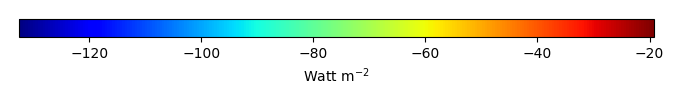MODEL MEANBIAS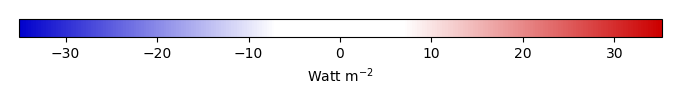BIAS SCORERMSE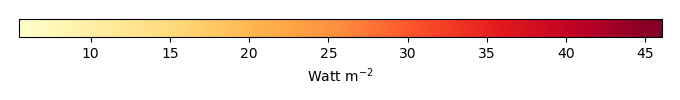RMSE SCOREBENCHMARK INTERANNUAL VARIABILITY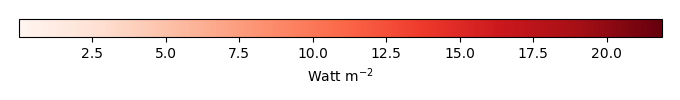MODEL INTERANNUAL VARIABILITYINTERANNUAL VARIABILITY SCOREBENCHMARK MAX MONTHMODEL MAX MONTHDIFFERENCE IN MAX MONTH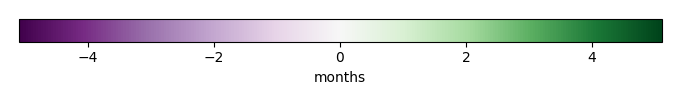SEASONAL CYCLE SCORESPATIAL TAYLOR DIAGRAMMODEL COLORS# Spatially integrated regional mean

MODEL COLORSREGIONAL MEANANNUAL CYCLEMONTHLY ANOMALYANNUAL CYCLE# All Models

BenchmarkCRUNCEPv7GSWP3v1WATCH# Data Information

creation_date: Tue Jul 1 08:25:00 PDT 2014

source_file: This product is generated from monthly 1 degree GEWEX SRB Radiation observations